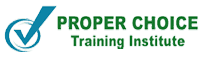# Types of Questions on Consecutive Integers

## Types of Questions Consecutive integers

Consecutive integers are the important part of SAT, ACT, GMAT and GRE Quantitative, based on this topic a variety of questions can be framed, some of those types will be discussed here. Before discussing about types of questions you may expect,let’shave a brief about consecutive integers.

What Consecutive integers are? Well, Consecutive integers are those numbers which follow one an other without skipping; those may be negative and positive including zero, examples are 7, 8, 9,… and ‐2, ‐1, 0, 1, 2, 3, 4, 5… are consecutive integers.

1. FINDING NUMBER OF TERMS IN GIVEN RANGE

Number of terms in a range, inclusive:(Last Term-First Term) +1

Number of terms between: (Last Term-First Term )-1

Example 1: How many integers are there from 5 to 10 inclusive?

Method: (Last Term – First Term )+1

For above example (10-5)+1=6

5,6,7,8,9,10

Number of multiples of ‘x’ in a given range: (Last multiple of x -First multiple of x)/ (Common difference)) + 1

Where x is an integer

Example 2: How many even numbers are there from 128 to 200 inclusive?

Solution: {(Last multiple of 2-First multiple of 2) ÷ 2} + 1

{(200-128)÷2} +1=37

• FINDING SUM OF CONSECUTIVE INTEGERS

Sum of terms: Average of terms *number of terms

Average of terms: (First term +Last term) /2

### Sum of Consecutive numbers in a range:  ((First term +Last term)/2 )*number of terms

Example 3: What is the sum of all integers from 80 to 120, inclusive?

Step 1: Find average of first and last term.

Average = (120+80) ÷2

=100

Step 2: Find the number of terms: 120-80+1= 41.

Step 3: Multiply the mean and number of terms 100×41=4100

Note

1. Sum of first n consecutive numbers is given by: n (n +1), Where n is the last term

2

1. Sum of first n positive even numbers= n (n +1), Where n is the number of even terms
2. Sum of first n positive odd numbers= n2, Where n is the number of odd terms
3. For any set of consecutive integers with an ODD number of items, the sum of all the integers is ALWAYS a multiple of the number of items.

Example 4:9+10+11+12+13=55

In the above example, number of items are 5(odd) so the sum 55, which is multiple of number of items

Students,who are willing to start SAT,ACTGMAT and GRE Preparation at Proper Choice Training Institute need not to worry about their basics of mathematics as we start coaching from the scratch to enhance their mathematical skills and prepare them to achieve their desired score.

Tags:

Share

Related Posts

Search

Enquiry Now

Recent posts